# 第 2 章 多臂老虎机

## 2.2 问题介绍

### 2.2.1 问题定义### 2.2.2 形式化描述

• 为动作集合，其中一个动作表示拉动一个拉杆。若多臂老虎机一共有根拉杆，那动作空间就是集合，我们用 表示任意一个动作；
• 为奖励概率分布，拉动每一根拉杆的动作都对应一个奖励概率分布，不同拉杆的奖励分布通常是不同的。

### 2.2.4 估计期望奖励

• 对于，初始化计数器 和期望奖励估值
• for do
• 选取某根拉杆，该动作记为
• 得到奖励
• 更新计数器:
• 更新期望奖励估值：
• end for

# 导入需要使用的库,其中numpy是支持数组和矩阵运算的科学计算库,而matplotlib是绘图库import numpy as npimport matplotlib.pyplot as plt

class BernoulliBandit:    """ 伯努利多臂老虎机,输入K表示拉杆个数 """    def __init__(self, K):        self.probs = np.random.uniform(size=K)  # 随机生成K个0～1的数,作为拉动每根拉杆的获奖        # 概率        self.best_idx = np.argmax(self.probs)  # 获奖概率最大的拉杆        self.best_prob = self.probs[self.best_idx]  # 最大的获奖概率        self.K = K
def step(self, k):        # 当玩家选择了k号拉杆后,根据拉动该老虎机的k号拉杆获得奖励的概率返回1（获奖）或0（未        # 获奖）        if np.random.rand() < self.probs[k]:            return 1        else:            return 0

np.random.seed(1)  # 设定随机种子,使实验具有可重复性K = 10bandit_10_arm = BernoulliBandit(K)print("随机生成了一个%d臂伯努利老虎机" % K)print("获奖概率最大的拉杆为%d号,其获奖概率为%.4f" %      (bandit_10_arm.best_idx, bandit_10_arm.best_prob))
随机生成了一个10臂伯努利老虎机获奖概率最大的拉杆为1号,其获奖概率为0.7203

class Solver:    """ 多臂老虎机算法基本框架 """    def __init__(self, bandit):        self.bandit = bandit        self.counts = np.zeros(self.bandit.K)  # 每根拉杆的尝试次数        self.regret = 0.  # 当前步的累积懊悔        self.actions = []  # 维护一个列表,记录每一步的动作        self.regrets = []  # 维护一个列表,记录每一步的累积懊悔
def update_regret(self, k):        # 计算累积懊悔并保存,k为本次动作选择的拉杆的编号        self.regret += self.bandit.best_prob - self.bandit.probs[k]        self.regrets.append(self.regret)
def run_one_step(self):        # 返回当前动作选择哪一根拉杆,由每个具体的策略实现        raise NotImplementedError
def run(self, num_steps):        # 运行一定次数,num_steps为总运行次数        for _ in range(num_steps):            k = self.run_one_step()            self.counts[k] += 1            self.actions.append(k)            self.update_regret(k)

## 2.4 ϵ-贪心算法

class EpsilonGreedy(Solver):    """ epsilon贪婪算法,继承Solver类 """    def __init__(self, bandit, epsilon=0.01, init_prob=1.0):        super(EpsilonGreedy, self).__init__(bandit)        self.epsilon = epsilon        #初始化拉动所有拉杆的期望奖励估值        self.estimates = np.array([init_prob] * self.bandit.K)
def run_one_step(self):        if np.random.random() < self.epsilon:            k = np.random.randint(0, self.bandit.K)  # 随机选择一根拉杆        else:            k = np.argmax(self.estimates)  # 选择期望奖励估值最大的拉杆        r = self.bandit.step(k)  # 得到本次动作的奖励        self.estimates[k] += 1. / (self.counts[k] + 1) * (r - self.estimates[k])        return k

def plot_results(solvers, solver_names):    """生成累积懊悔随时间变化的图像。输入solvers是一个列表,列表中的每个元素是一种特定的策略。    而solver_names也是一个列表,存储每个策略的名称"""    for idx, solver in enumerate(solvers):        time_list = range(len(solver.regrets))        plt.plot(time_list, solver.regrets, label=solver_names[idx])    plt.xlabel('Time steps')    plt.ylabel('Cumulative regrets')    plt.title('%d-armed bandit' % solvers.bandit.K)    plt.legend()    plt.show()

np.random.seed(1)epsilon_greedy_solver = EpsilonGreedy(bandit_10_arm, epsilon=0.01)epsilon_greedy_solver.run(5000)print('epsilon-贪婪算法的累积懊悔为：', epsilon_greedy_solver.regret)plot_results([epsilon_greedy_solver], ["EpsilonGreedy"])
psilon-贪婪算法的累积懊悔为：25.526630933945313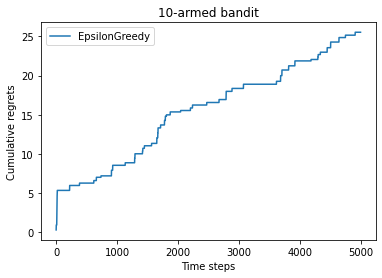np.random.seed(0)epsilons = [1e-4, 0.01, 0.1, 0.25, 0.5]epsilon_greedy_solver_list = [    EpsilonGreedy(bandit_10_arm, epsilon=e) for e in epsilons]epsilon_greedy_solver_names = ["epsilon={}".format(e) for e in epsilons]for solver in epsilon_greedy_solver_list:    solver.run(5000)
plot_results(epsilon_greedy_solver_list, epsilon_greedy_solver_names)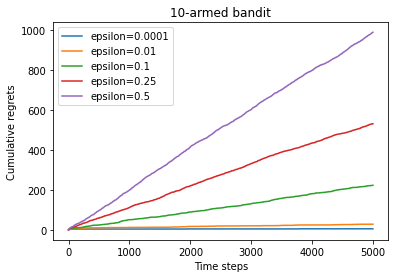class DecayingEpsilonGreedy(Solver):    """ epsilon值随时间衰减的epsilon-贪婪算法,继承Solver类 """    def __init__(self, bandit, init_prob=1.0):        super(DecayingEpsilonGreedy, self).__init__(bandit)        self.estimates = np.array([init_prob] * self.bandit.K)        self.total_count = 0
def run_one_step(self):        self.total_count += 1        if np.random.random() < 1 / self.total_count:  # epsilon值随时间衰减            k = np.random.randint(0, self.bandit.K)        else:            k = np.argmax(self.estimates)
r = self.bandit.step(k)        self.estimates[k] += 1. / (self.counts[k] + 1) * (r - self.estimates[k])
return k

np.random.seed(1)decaying_epsilon_greedy_solver = DecayingEpsilonGreedy(bandit_10_arm)decaying_epsilon_greedy_solver.run(5000)print('epsilon值衰减的贪婪算法的累积懊悔为：', decaying_epsilon_greedy_solver.regret)plot_results([decaying_epsilon_greedy_solver], ["DecayingEpsilonGreedy"])
epsilon值衰减的贪婪算法的累积懊悔为：10.114334931260183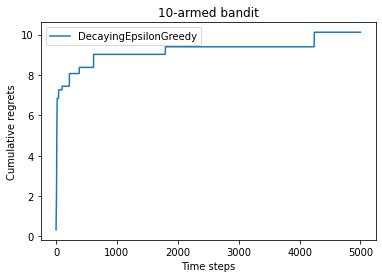## 2.5 上置信界算法

class UCB(Solver):    """ UCB算法,继承Solver类 """    def __init__(self, bandit, coef, init_prob=1.0):        super(UCB, self).__init__(bandit)        self.total_count = 0        self.estimates = np.array([init_prob] * self.bandit.K)        self.coef = coef
def run_one_step(self):        self.total_count += 1        ucb = self.estimates + self.coef * np.sqrt(            np.log(self.total_count) / (2 * (self.counts + 1)))  # 计算上置信界        k = np.argmax(ucb)  # 选出上置信界最大的拉杆        r = self.bandit.step(k)        self.estimates[k] += 1. / (self.counts[k] + 1) * (r - self.estimates[k])        return k

np.random.seed(1)coef = 1  # 控制不确定性比重的系数UCB_solver = UCB(bandit_10_arm, coef)UCB_solver.run(5000)print('上置信界算法的累积懊悔为：', UCB_solver.regret)plot_results([UCB_solver], ["UCB"])
上置信界算法的累积懊悔为： 70.45281214197854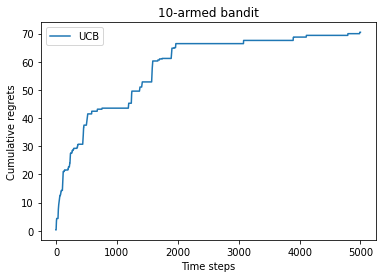## 2.6 汤普森采样算法

MAB 中还有一种经典算法——汤普森采样（Thompson sampling），先假设拉动每根拉杆的奖励服从一个特定的概率分布，然后根据拉动每根拉杆的期望奖励来进行选择。但是由于计算所有拉杆的期望奖励的代价比较高，汤普森采样算法使用采样的方式，即根据当前每个动作 的奖励概率分布进行一轮采样，得到一组各根拉杆的奖励样本，再选择样本中奖励最大的动作。可以看出，汤普森采样是一种计算所有拉杆的最高奖励概率的蒙特卡洛采样方法。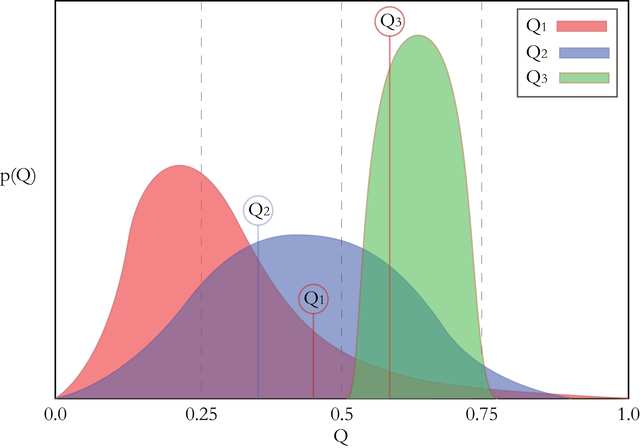class ThompsonSampling(Solver):    """ 汤普森采样算法,继承Solver类 """    def __init__(self, bandit):        super(ThompsonSampling, self).__init__(bandit)        self._a = np.ones(self.bandit.K)  # 列表,表示每根拉杆奖励为1的次数        self._b = np.ones(self.bandit.K)  # 列表,表示每根拉杆奖励为0的次数
def run_one_step(self):        samples = np.random.beta(self._a, self._b)  # 按照Beta分布采样一组奖励样本        k = np.argmax(samples)  # 选出采样奖励最大的拉杆        r = self.bandit.step(k)
self._a[k] += r  # 更新Beta分布的第一个参数        self._b[k] += (1 - r)  # 更新Beta分布的第二个参数        return k

np.random.seed(1)thompson_sampling_solver = ThompsonSampling(bandit_10_arm)thompson_sampling_solver.run(5000)print('汤普森采样算法的累积懊悔为：', thompson_sampling_solver.regret)plot_results([thompson_sampling_solver], ["ThompsonSampling"])
汤普森采样算法的累积懊悔为：57.19161964443925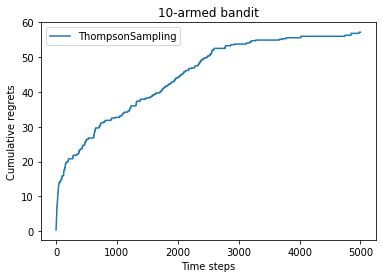## 2.8 参考文献

 AUER P, CESA-BIANCHI N,FISCHER P. Finite-time analysis of the multiarmed bandit problem[J]. Machine learning,2002, 47 (2) : 235-256.

 AUER P. Using confidence bounds for exploitation-exploration trade-offs[J]. Journal of Machine Learning Research,2002, 3(3): 397-422.

 GITTINS J, GLAZEBROOK K,WEBER R. Multi-armed bandit allocation indices[M]. 2nd ed. America: John Wiley & Sons, 2011.

 CHAPELLE O, LI L. An empirical evaluation of thompson sampling [J]. Advances in neural information processing systems, 2011, 24: 2249-2257.